# BITSAT Mathematics Statistics MCQs

BITSAT Mathematics Statistics MCQs with answers available in Pdf for free download. The MCQ Questions for BITSAT Mathematics with answers have been prepared as per the latest BITSAT Mathematics syllabus, books and examination pattern. Multiple Choice Questions form important part of competitive exams and BITSAT exam and if practiced properly can help you to get higher rank. Refer to more topic wise BITSAT Mathematics Questions and also download more latest study material for all subjects and do free BITSAT Mathematics Mock Test

## MCQ for BITSAT Mathematics Statistics

BITSAT Mathematics students should refer to the following multiple-choice questions with answers for Statistics in BITSAT. These MCQ questions with answers for BITSAT Mathematics will come in exams and help you to score good marks

### Statistics MCQ Questions with Answers

#### Question: The marks obtained by 60 students in a certain test are given below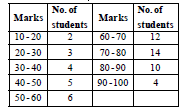Median of the above data is

• a) 68.33
• b) 70
• c) 68.11
• d) None of these

#### Question: The arithmetic mean of numbers a, b, c, d, e is M. What is the value of (a – M) + (b – M) + (c – M)+ (d – M) + (e – M) ?

• a) M
• b) a + b + c + d + e
• c) 0
• d) 5 M

#### Question: The arithmetic mean of the data 0, 1, 2, ......, n with frequencies 1, nC1, nC2,....., nCn is

• a) n
• b)• c) n + 1
• d)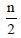#### Question: The mean square deviation of a set of n observation x1, x2, .... xn about a point c is defined asThe mean square deviations about – 2 and 2 are 18 and 10 respectively, the standard deviation of this set of observations is

• a) 3
• b) 2
• c) 1
• d) None of these

#### Question: If M. D. is 12, the value of S.D. will be

• a) 15
• b) 12
• c) 24
• d) None of these

• a) 70
• b) 64
• c) 90
• d) 50

#### Question: Find the variance of the data given below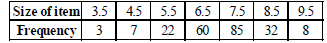• a) 1.29
• b) 2.19
• c) 1.32
• d) None of these

• a) 1
• b) 9/4
• c) 4/9
• d) 2/3

• a) 10
• b) 20
• c) 15
• d) 25

#### Question: Mean of 25 observations was found to be 78.4. But later on it was found that 96 was misread 69. The correct mean is

• a) 79.24
• b) 79.48
• c) 80.10
• d) None of these

#### Question: If the mean, mode and S.D. of a frequency distribution are 41,45 and 8 respectively, then its Pearson’s coefficient of skewness is

• a)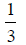• b)• c)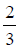• d) 1#### Question: Given below is a frequency distribution with median 46. In this distribution, some of the frequencies are missing : Determine the missing frequencies.• a) 34, 45
• b) 25, 40
• c) 12, 18
• d) 30, 35

• a) 5.113
• b) 4.606
• c) 4.134
• d) 4.909

• a) 4.5
• b) 13.5
• c) 9
• d) 3.5